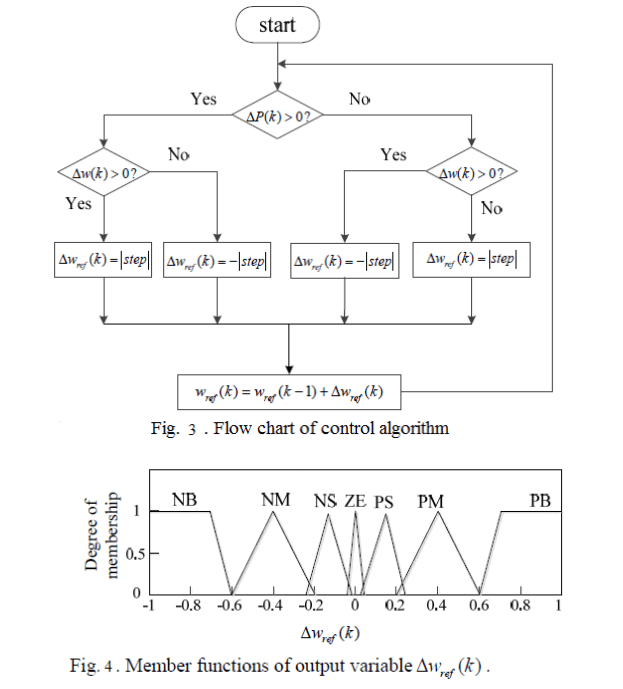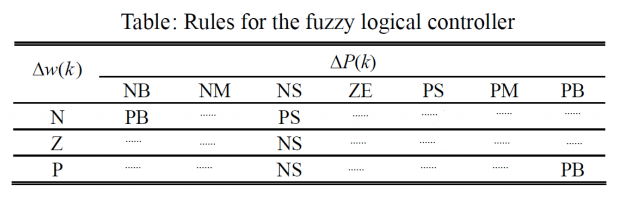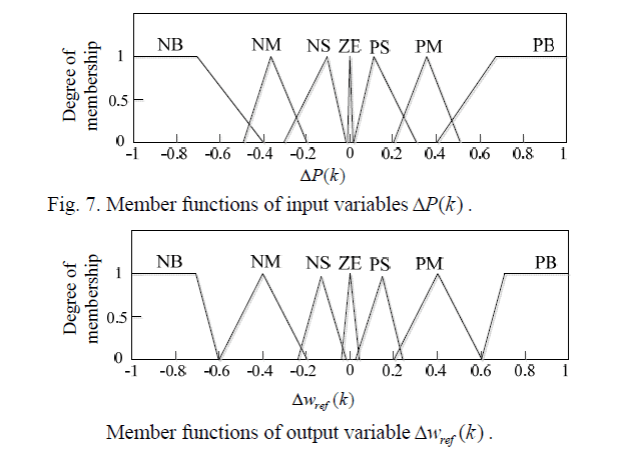# MPPT-Wind turbine

EXERCISE: MAXIMUM POWER POINT TRACKER FOR A WIND TURBINE

Maximum Power Point Tracking (MPPT), or MPP controller or MPP tracker is a principle to follow the maximum power point of a nonlinear electrical generator. MPPT systems are generally associated with PV or wind generators. We give you some illustrations of an MPPT control for a wind turbine with a permanent magnet synchronous machine.We propose to develop a fuzzy MPPT controller for the wind turbine.
1) Explain the principle of proposed MPPT method.
2) Well define inputs and output.
3) Draw inputs and output membership functions with notations (such as N, Z, P or NB, NS, NM, PB, PM, PS, ZE).

We suppose normalized variables with values between -1 and 1.
4) Cite at least five if then rules as example.

5) Complete this fuzzy logic rules table (Fuzzy Associative Matrix)CORRECTION :

1) The basic principle of the Hill Climbing searching algorithm is : if the previous increment of rotational speed ∆w results in an increase of mechanical power ∆P, the search of ∆w continues in the same direction ; otherwise, the search reverses its direction.

2) The set of fuzzy logical controller is described as follows: The input variables are ΔP(k) and Δw(k), while the output variable is  Δwref(k). ΔP(k) and Δw(k) can be obtained by:      ΔP(k)=P(k) -P(k-1)  and   Δw(k) = w(k) – w(k-1).

3)4)

If ∆w is N and ∆P is NB then ∆wref is PB
If ∆w is N and ∆P is NS then ∆wref is PS
If ∆w is Z and ∆P is NS then ∆wref is NS
If ∆w is P and ∆P is NS then ∆wref is NS
If ∆w is P and ∆P is PB then ∆wref is PB

5)